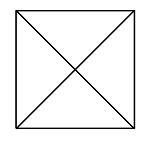# Calculation of the pyramid (Sequence of numerical progressions).

## Recommended Posts

Front side:Progress Sums:-1,-2,-3,-4,-5... 1,2,3,4,5...

We express the formulas:Sn= (a₁n2+n)/2, ; Sn-1=(a₁n2-n)/2,. (n - Number of summing members, a₁ - first member of the progression. With a negative or positive value n. Expressions Sn-1, Sn-2 should be understood: subtraction from the number of the member taken).

First option:

Example: Sn= (a₁n2+n)/2.

For n = -5 we have: (-1*(-5)2+(-5))/2=-15; For n = 5 we have: (1*(5)2+5)/2=15.

Example: Sn-1=(a₁n2-n)/2

For n = -5 we have: (-1*(-5)2-(-5))/2=-10 For n = 5 we have: (1*(5)2-5)/2=10.

Triangular:Progress Sums:-1,-3,-6,-10,-15....1,3,6,10,15....

We express the formulas:Sn= ((n+a₁)3-(n+a₁))/6, Sn= (n3-n)/6+(a₁n2+n)/2; Sn-1=(n3-n)/6; Sn-2=((n-a₁)3-(n-a₁))/6, Sn-2=(n3-n)/6-(a₁n2-n)/2.

First option:

Example: Sn= ((n+a₁)3-(n+a₁))/6.

For n = -5 we have: ((-5+(-1))3-(-5+(-1)))/6=-35; For n = 5 we have: ((5+1)3-(5+1))/6=35.

Example: Sn-2=((n-a₁)3-(n-a₁))/6.

For n = -5 we have:((-5-(-1))3-(-5-(-1)))/6=-10; For n = 5 we have: ((5-1)3-(5-1))/6=10.

Second option:

Example: Sn= (n3-n)/6+(a₁n2+n)/2.

For n = -5 we have: ((-5)3-(-5))/6+(-1*(-5)2+(-5))/2= -35; For n = 5 we have: (( 5)3-5)/6+(1*(5)2+5)/2= 35.

Example: Sn-1=(n3-n)/6.

For n = -5 we have: ((-5)3-(-5))/6= -20; For n = 5 we have: (( 5)3-5)/6=20.

Example: Sn-2=(n3-n)/6-(a₁n2-n)/2.

For n = -5 we have:((-5)3-(-5))/6 -(-1*(-5)2-(-5))/2= -10; For n = 5 we have: (( 5)3-5)/6-(1*(5)2-5)/2= 10.Progress Sums: -1,-4,-9,-16,-25....1,4,9,16,25....

We express the formulas:Sn= a₁(n+a₁)(a₁n2+0,5n)/3, Sn= (n3-n)/3 + (a₁n2+n)/2; Sn-1= a₁(n-a₁)(a₁n2-0,5n)/3, Sn-1=(n3-n)/3 - (a₁n2-n)/2.

First option:

Example: Sn=a₁(n+a₁)(a₁n2+0,5n)/3.

For n = -5 we have: -1(-5+(-1))*(-1*(-5)2+(-2,5))/3=-55; For n = 5 we have: 1(5+1)(1*(5)2+2,5)/3=55.

Example: Sn-1= a₁(n-a₁)(a₁n2-0,5n)/3.

For n = -5 we have: -1(-5-(-1))*(-1*(-5)2-(-2,5))/3=-30; For n = 5 we have:1(5-1)(1*(5)2-2,5)/3=30.

Second option:

Example: Sn= (n3-n)/3 + (a₁n2+n)/2.

For n = -5 we have: ((-5)3-(-5))/3 + (-1*(-5)2+(-5))/2= -55; For n = 5 we have:((5)3-5)/3 + (1*(5)2+5)/2= 55.

Example: Sn-1= (n3-n)/3 -(a₁n2-n)/2.

For n = -5 we have: ((-5)3-(-5))/3 -(-1*(-5)2-(-5))/2= -30; For n = 5 we have: ((5)3-5)/3 -(1*(5)2-5)/2= 30.

Edited by Andrei_62
##### Share on other sites

!

Moderator Note

Is there a question here, or a direction for the discussion?

##### Share on other sites

Pyramids are 3-dimensional; polygonal numbers are 2-dimensional. They are not interchangeable.

## Create an account

Register a new account